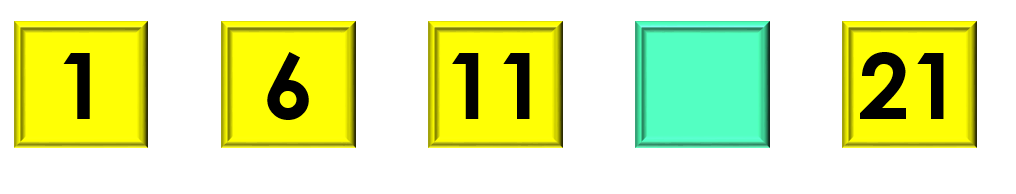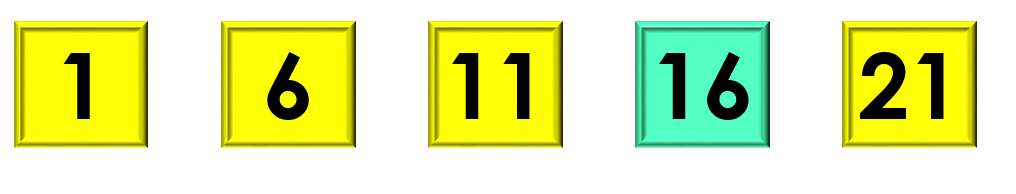1
visibility

Consider the given number pattern and identify the missing number.• A

26

• B

16

• C

17

• D

22

Solution:

Considering the given number pattern.Here, the numbers are increasing.
So, to find the missing number, we first find the rule.

Note that:
1 + 5 = 6
6 + 5 = 11
11 + 5 = 16
16 + 5 = 21

So, the rule is "add 5".Then, the missing number is 16.